# ELM Test - Geometry: Graphing Basics Chapter Exam

Exam Instructions:

Choose your answers to the questions and click 'Next' to see the next set of questions. You can skip questions if you would like and come back to them later with the yellow "Go To First Skipped Question" button. When you have completed the practice exam, a green submit button will appear. Click it to see your results. Good luck!

### Page 1

#### Question 2 2. Which point is identified on this graph?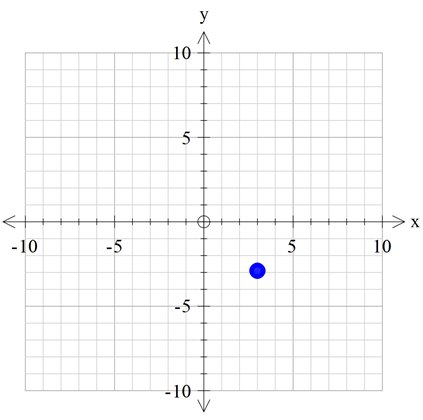### Page 2

#### Question 6 6. Use the number line to solve -5 - 2 + 3.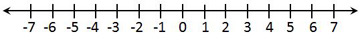#### Question 7 7. What is the name of the point located in this graph?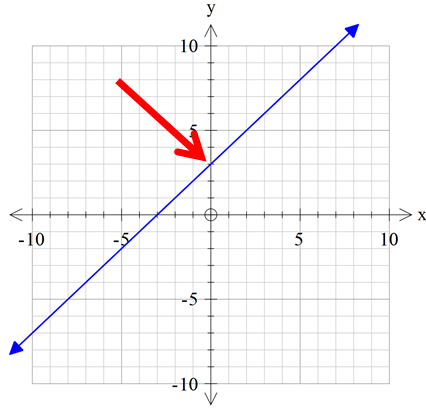#### Question 9 9. What is the maximum value on this number line?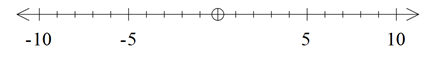#### Question 10 10. What number does the point on this line represent?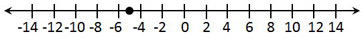### Page 3

#### Question 15 15. In which quadrant is the point (3, 6)?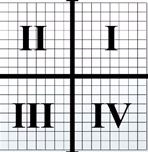### Page 4

#### Question 16 16. The following graph displays which point?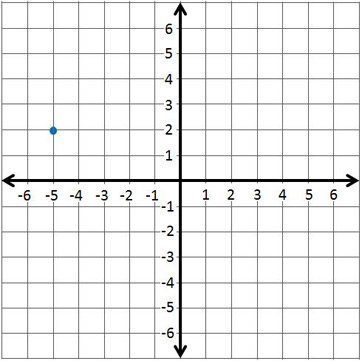#### Question 17 17. Use the number line to solve -4 + 6 - 3 + 7.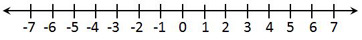#### Question 18 18. What value does the point represnt on the number line?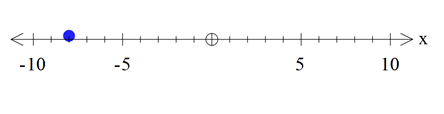#### Question 19 19. The following graph displays which point?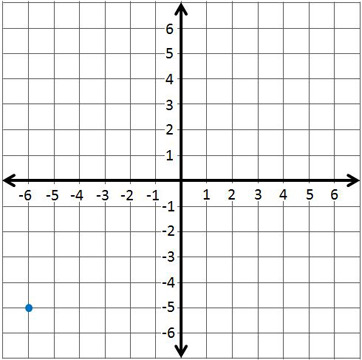### Page 5

#### Question 22 22. Each mark on this number line represents what value?#### Question 24 24. In which quadrant is the point (-2, 5)?#### Question 25 25. The following graph displays which point?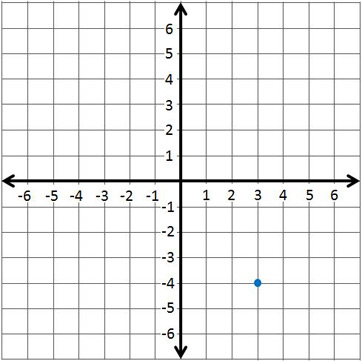### Page 6

#### Question 26 26. Use the number line to solve 9 - 13 + 7 - 3.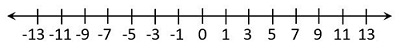#### Question 27 27. What is the name of the line pointed to in the this coordinate plane?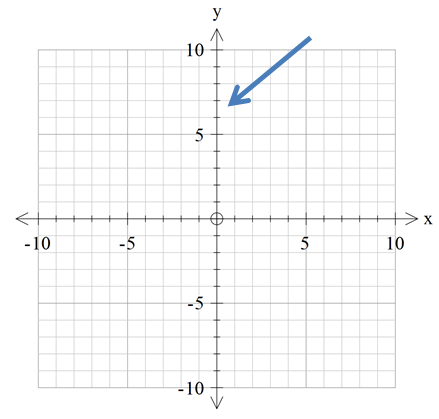#### Question 28 28. What are the coordinates of this point?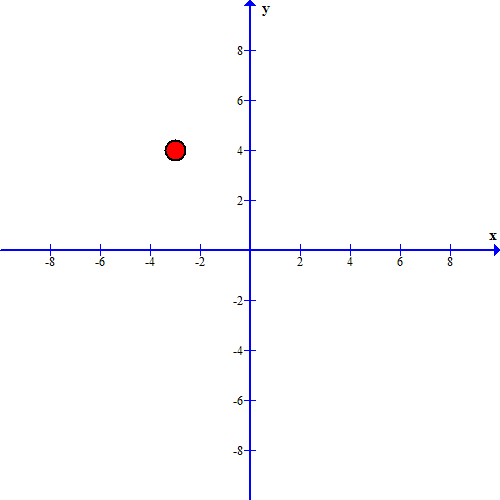#### ELM Test - Geometry: Graphing Basics Chapter Exam Instructions

Choose your answers to the questions and click 'Next' to see the next set of questions. You can skip questions if you would like and come back to them later with the yellow "Go To First Skipped Question" button. When you have completed the practice exam, a green submit button will appear. Click it to see your results. Good luck!

Support